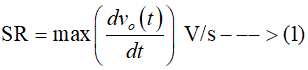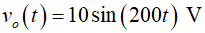# The output voltage of an opamp is v(t)=10sin(200t) V. Find out the value of the slew rate of the opamp.

Question
1 views

The output voltage of an opamp is v(t)=10sin(200t) V.

Find out the value of the slew rate of the opamp.

check_circle

star
star
star
star
star
1 Rating
Step 1

The slew rate (SR) of an operational amplifier (opamp) is defined as the maximum rate of change in the output voltage (vo) signal. It can be expressed asStep 2

In this case, the output voltage is given as...

### Want to see the full answer?

See Solution

#### Want to see this answer and more?

Solutions are written by subject experts who are available 24/7. Questions are typically answered within 1 hour.*

See Solution
*Response times may vary by subject and question.
Tagged in